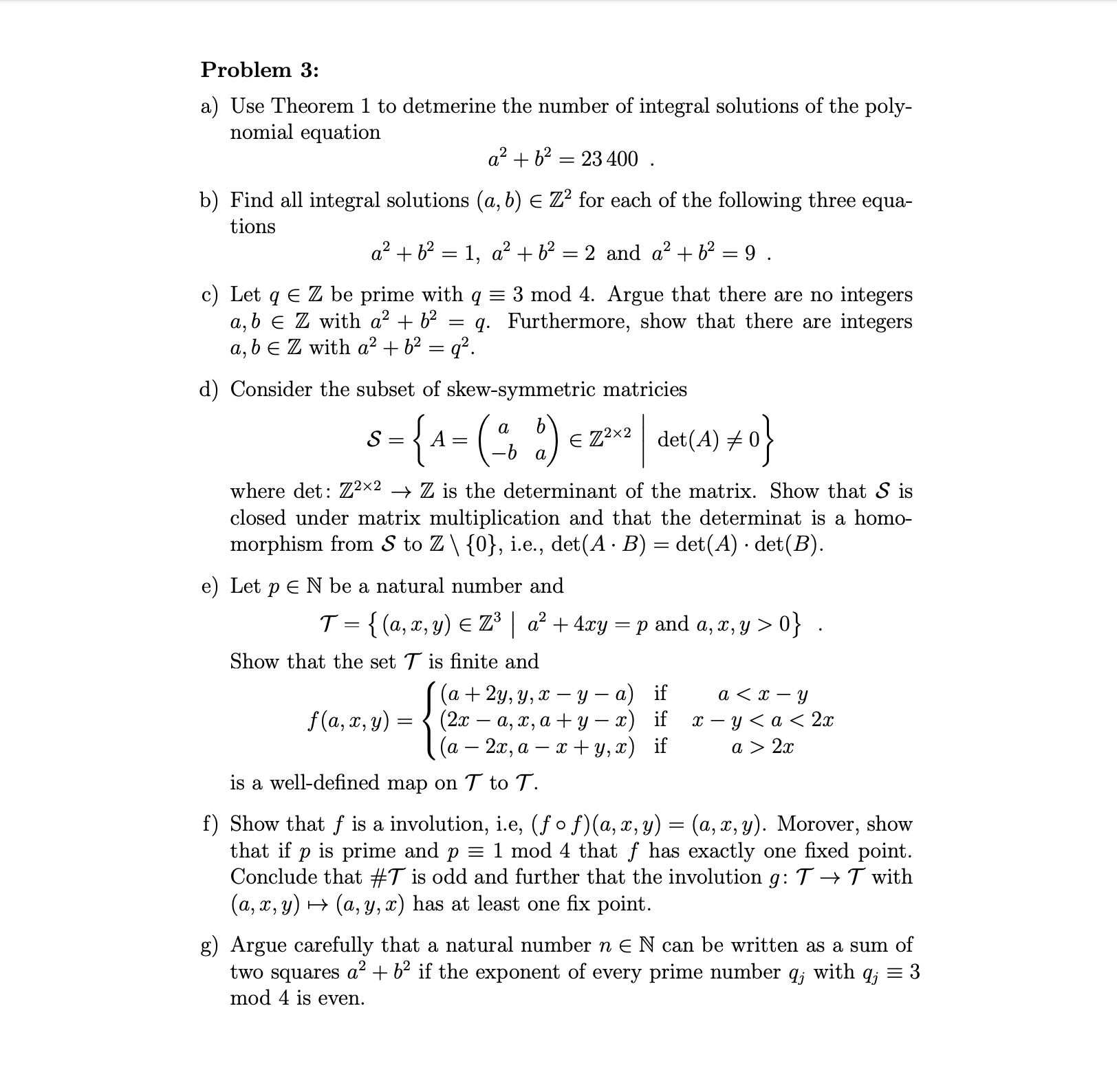# Problem 3:a) Use Theorem 1 to detmerine the number of integral solutions of the poly-nomial equationa2b22340011b) Find all integral solutions (a, b) E Z2 for each of the following three equa-tionsa2b21, a2 b22 and a2b2 =9 .c) Let q E Z be prime with qa, b E Z with a2 + b2a, bE Z with a2b2 = q23 mod 4. Argue that there are no integersq. Furthermore, show that there are integersd) Consider the subset of skew-symmetric matricies8{A=(2)e Z2x2det(A)Sawhere det: Zx2 > Z is the determinant of the matrix. Show that S isclosed under matrix multiplication and that the determinat is a homo-morphism from S to Z\ {0}, i.e., det(A B) det(A) det(B)e) Let pE N be a natural number andT {(a,x, y) E Z3a2 + 4xry p and a, z, y > 0} .Show that the set T is finite and(а + 2у, у, х — у — а) if(2х — а, х, а + у — 2) if(a 2x, a y, x) iff(a, x, y)х — у 2xis a well-defined map on T to T.f) Show that f is a involution, i.e, (fo f)(a, x, y) (a, x, y). Morover, showthat if p is prime and p = 1 mod 4 that f has exactly one fixed pointConclude that #T is odd and further that the involution g: T ->T with(a, x, y)(a, y, x) has at least one fix point.g) Argue carefully that a natural number n E N can be written as a sum oftwo squares a +b2 if the exponent of every prime number qj with qj = 3mod 4 is even.

Question
61 views

Problem 3 part bhelp_outlineImage TranscriptioncloseProblem 3: a) Use Theorem 1 to detmerine the number of integral solutions of the poly- nomial equation a2b223400 11 b) Find all integral solutions (a, b) E Z2 for each of the following three equa- tions a2b21, a2 b2 2 and a2b2 =9 . c) Let q E Z be prime with q a, b E Z with a2 + b2 a, bE Z with a2b2 = q2 3 mod 4. Argue that there are no integers q. Furthermore, show that there are integers d) Consider the subset of skew-symmetric matricies 8{A=(2) e Z2x2det(A) S a where det: Zx2 > Z is the determinant of the matrix. Show that S is closed under matrix multiplication and that the determinat is a homo- morphism from S to Z\ {0}, i.e., det(A B) det(A) det(B) e) Let pE N be a natural number and T {(a,x, y) E Z3a2 + 4xry p and a, z, y > 0} . Show that the set T is finite and (а + 2у, у, х — у — а) if (2х — а, х, а + у — 2) if (a 2x, a y, x) if f(a, x, y) х — у <а< 2x a > 2x is a well-defined map on T to T. f) Show that f is a involution, i.e, (fo f)(a, x, y) (a, x, y). Morover, show that if p is prime and p = 1 mod 4 that f has exactly one fixed point Conclude that #T is odd and further that the involution g: T ->T with (a, x, y)(a, y, x) has at least one fix point. g) Argue carefully that a natural number n E N can be written as a sum of two squares a +b2 if the exponent of every prime number qj with qj = 3 mod 4 is even. fullscreen
check_circle

Step 1

(1)

consider the first equation:

Step 2

Now, since a2 and b2 are always greater than or equal to zero that is:

Step 3

So,

Here, the possible integral solutions (a, b) for the first equatio...

### Want to see the full answer?

See Solution

#### Want to see this answer and more?

Solutions are written by subject experts who are available 24/7. Questions are typically answered within 1 hour.*

See Solution
*Response times may vary by subject and question.
Tagged in

### Math